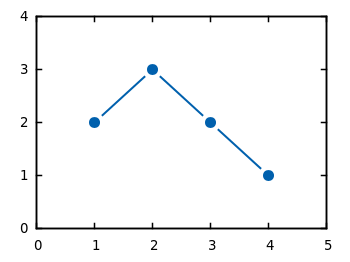# Gnuplotting

Create scientific plots using gnuplot

August 13th, 2010 | 7 Comments

In Gnuplot it is easy to define a continuous and differentiable function such as `f(x) = x`, but what to do if we need a function that fulfill non of these conditions?
For example let us consider a step function. Typically a step function is given by

```           / 1   if x > a
step(x) = -|
\ 0   else
```

In Gnuplot this can be achieved by using the ternary operator:

```step(x) = x>a ? 1 : 0
```

Which is a simple if-else statement and means `step(x)=1 if x>a else step(x)=0`.

If we plot this function we get Fig. 1.

Fig. 1 Continuous plot of the step function (code to produce this figure)

As you can see this will result in a continuous plot. If we want a discontinuity in the plot, we have to create two separate functions that are only piecewise defined. This can be achieved by using `1/0` that will result in a undefined value.

```f(x) = x<a  ? 1 : 1/0
g(x) = x>=a ? 1 : 1/0
```

Plotting both functions will result in Fig. 2.

Fig. 2 Discontinuous plot of the step function (code to produce this figure)

The ternary operator can also be used in an iterative way. For example if we want to define a rectangular function that is given by

```           / 0     if abs(x) > 0.5
rect(x) = -| 0.5   if abs(x) = 0.5
\ 1     if abs(x) < 0.5
```

we can use the following statement in Gnuplot to define it:

```rect(x) = abs(x)>0.5 ? 0 : abs(x)<0.5 ? 1 : 0.5
```

In Fig. 3 you can see a plot of this function. To produce the sharp edges of the rectangular function we use a higher number of sampling points (also in Fig. 1 for the step function). In order to plot a function Gnuplot calculates 100 points of the given function and draw a line through them. This can be set to another value with the `set samples <value>` command.

Fig. 3 Plot of the above defined rect(x) function (code to produce this figure)

June 9th, 2010 | 11 Comments

If you have measurement data and like to plot them as points combined by lines, you will probably do that with the `linespoints` plotting style. But for some applications it is required to combine the data points by non-continuous lines to emphasize that the data came from measurements as shown in Fig. 1.Fig. 1 Plot of the data from plotting_data1.dat with non-coninuous lines between its points (code to produce this figure)

In Gnuplot exists no line style that can do this directly. But with a little trick it is very easy to achieve. Since Gnuplot 4.4. there exists the property `pointinterval` (see the documentation) in combination with the plotting style ` linespoints`. This property plots not every single point, but only every second for a value of `2` and so on. But if we use the value `-1` it tells Gnuplot to insert a little gap between the points and the line. The size of the gap can be set by the `pointintervalbox` property.

```set style line 1 lc rgb '#0060ad' lt 1 lw 2 pt 7 pi -1 ps 1.5
set pointintervalbox 3
```

We specify a point interval `pi` of -1 and a point size of 1.5, in addition we set the the gap to a point size of 3. Now we can plot our data with the `linespoints` style.

```plot 'plotting_data1.dat' with linespoints ls 1
```

Using the same data as in the first plot of the gnuplot basics tutorial Plotting data we will get Fig. 1 as a result.